• No products in the cart.

# 203.7.7 Boosting

##### Concepts behind Boosting.

In previous section, we studied about Random Forest

In this post we will cover how boosting work and the type of boosting algorithms.

### Boosting

• Boosting is one more famous ensemble method.
• Boosting uses a slightly different techniques to that of bagging.
• Boosting is a well proven theory that works really well on many of the machine learning problems like speech recognition.
• If bagging is wisdom of crowds then boosting is wisdom of crowds where each individual is given some weight based on their expertise.
• Boosting in general decreases the bias error and builds strong predictive models.
• Boosting is an iterative technique. We adjust the weight of the observation based on the previous classification.
• If an observation was classified incorrectly, it tries to increase the weight of this observation and vice versa.

### Boosting Main idea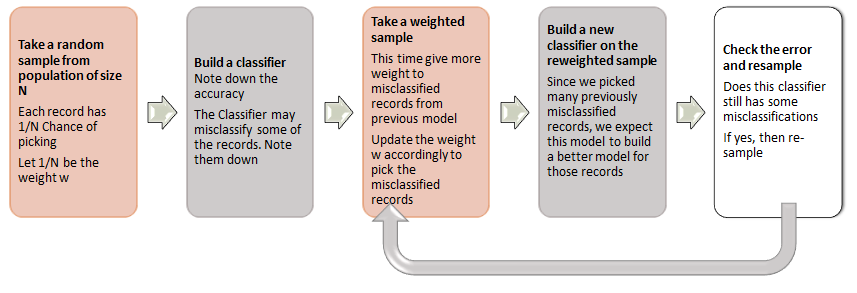Final Classifier $$C = \sum \alpha_i c_i$$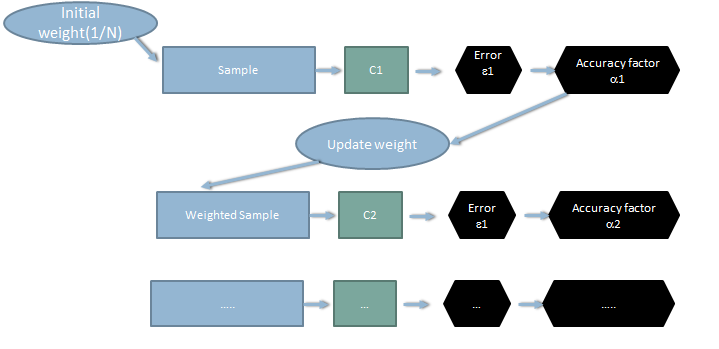### How weighted samples are taken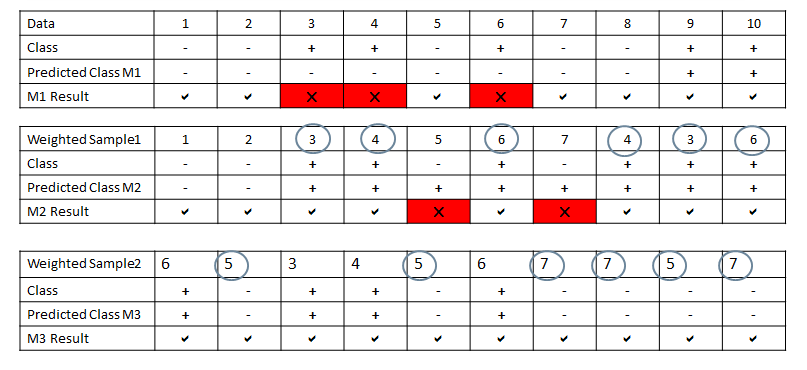### Boosting Illustration

Below is the training data and their classes .We need to take a note of record numbers, they will help us in weighted sampling later.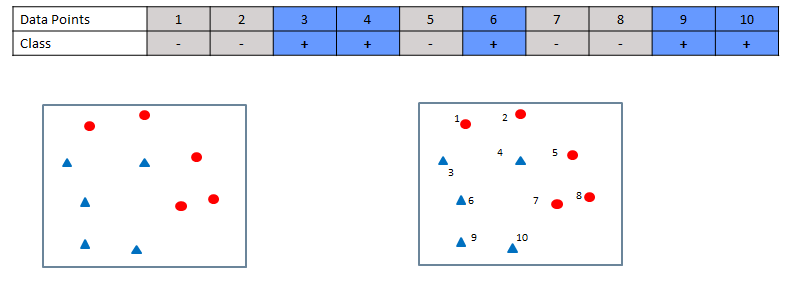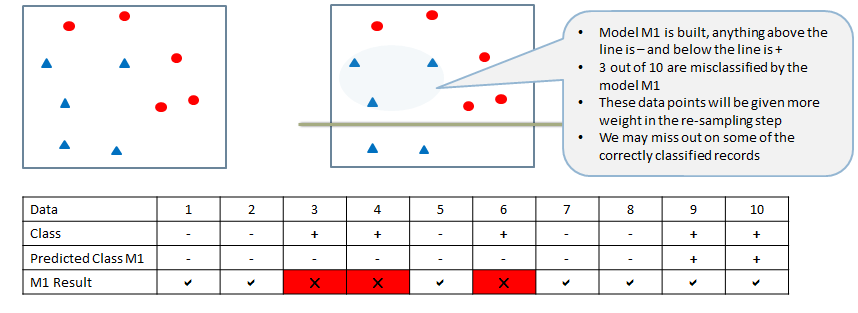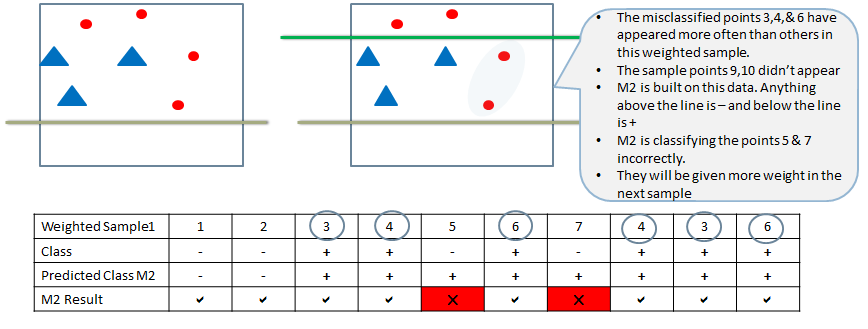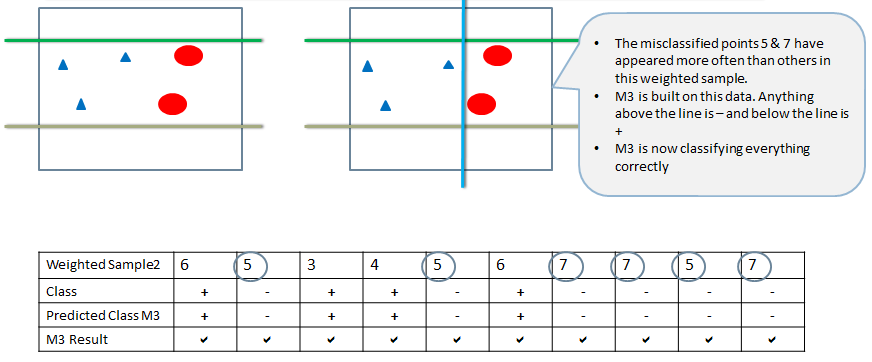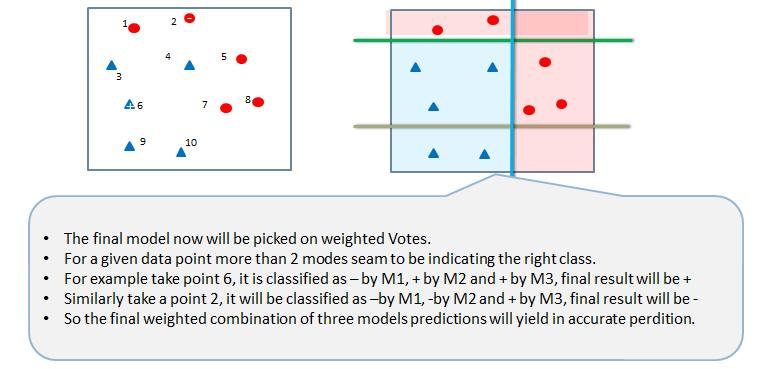### Theory behind Boosting Algorithm

• Take the dataset
• Build a classifier $$C_m$$ and find the error
• Calculate error rate of the classifier
• Error rate of $$\epsilon _m = \sum w_i I (y_i \neq C_m (x)) / \sum w_i$$ = Sum of misclassification weight / sum of sample weights
• Calculate an intermediate factor called a. It analogous to accuracy rate of the model. It will be later used in weight updating. It is derived from error
• $$\alpha _m = log(1- \epsilon _m)/\epsilon _m)$$
• Update weights of each record in the sample using the a factor. The indicator function will make sure that the misclassifications are given more weight
• For i =1,2,… N
• $$W_(i+1) = w_i e^(\alpha _m I(y_i\neq C_m (x)))$$
• Renormalize so that sum of weights is 1
• Repeat this model building and weight update process until we have no misclassification
• Final collation is done by voting from all the modes. While taking the votes, each model is weighted by the accuracy factor $$\alpha$$
• $$C = sign(\sum \alpha _i C_i(x))$$